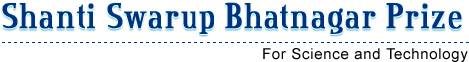• |• Home
• Brief Profile of the Awardee

## Brief Profile of the Awardee## DrAmlendu Krishna

• 2016
• Mathematical Sciences
• 02/08/1971
• Algebraic Cycles, Algebraic K-Theory, Algebraic Geometry
##### Award Citation:

Dr Krishna has made outstanding contributions to K-theory, proof of analogue of Atiyah- Segal completion theorem and a solution of Bloch-Srinivas conjecture.

 NA
 NA
 NA
 NA
##### (a) Significant contributions to science and/ or technology development by the nominee based on the work done in India during most part of last 5 years:
The work done by the nominee during the 5-year period prior to this year are divided into the following three broad topics. 1. Algebraic cycles on singular schemes: Beginning with the time of Chow and then Grothendieck , the theory of Chow groups of smooth algebraic varieties has been known as the most powerful cohomology theory for smooth algebraic varieties defined over a field. It is known that most of the other cohomology theories of such varieties can be realized from their Chow groups. However, this cohomology theory of algebraic varieties has a serious drawback. The problem is that the theory of Chow groups FAIL to define a cohomology theory for algebraic varieties, which have singularities. In complex geometry, these varieties occur as those analytic spaces which do not form a complex manifold. Such varieties are ubiquitous in algebraic and complex geometry. There has been several attempts worldwide to define an algebraic cohomology theory for singular varieties. But all attempts have so far failed. In one such attempt, Levine and Weibel invented the theory of Chow group of 0-cycles on singular varieties. This Chow group of 0-cycles has since then been extensively studied by Srinivas, Levine, Weibel and others with remarkable success. Conjecture of Bloch and Srinivas: Around 1982, Bloch and Srinivas gave a conjectural explicit description of the Chow group of 0-cycles on a normal surface \$X\$ in terms of a relative Chow groups of 0-cycles on the resolution of singularities of \$X\$. This conjecture was affirmatively solved by Amalendu in collaboration with V. Srinivas and the solution appears in the reference (1 ). Several outstanding applications of th is solutions were also obtained in (1 ). It was already observed long ago that the conjecture of Bloch and Srinivas can be formulated for singular varieties of any dimension. Amalendu worked on this generalized Bloch-Srinivas conjecture for few years and wrote (3) and (4). This work culminated in (11 ), where he completely solved the generalized Bloch-Srinivas conjecture for all varieties with isolated Cohen-Macaulay singularities in characteristic zero. Building on his work, Matthew Morrow has been able to remove the condition of isolated singularities in last one year. As a result, Bloch-Srinivas conjecture is now reasonably settled in characteristic zero. In one of his most recent works, Amalendu has used his solution to the BlochSrinivas conjecture to give an explicit description of the class field theory of function fields of varieties over a finite field in terms of the Chow group of 0-cycles on singular schemes . This has led to simple proofs of some hard results and Moritz Kerz and Shuji Saito for a class of smooth varieties with no restriction on the characteristic of the ground field. These results solve a conjecture of Deligne in number theory. Amalendu has also used these results to solve the Bloch-Srinivas conjecture for projective varieties over finite fields which have isolated singularities. In another recent work on the subject of K-theory, Amalendu has affirmatively answered a long term open problem of P. Murthy. This problem asks if the Chow group of 0-cycles on affine varieties of dimension at least two over an algebraically closed field is torsion free.
##### (b) Impact of the contributions in the field concerned:
The Bloch-Srinivas conjecture has now been verified in all dimensions in characteristic zero. This has led to a very good understanding of the theory of 0-cycles on singular varieties. The equivariant Riemann-Roch theorem has led to the confirmation that the equivariant Chow groups of Edidin-Graham have expected properties of equivariant motivic cohomology theory. It provides very efficient tools to compute equivariant K-theory. The algebraic Atiyah-Segal theorem of Amalendu allows a strong tool to compute the equivariant K-theory of algebraic varieties.
 NA
 NA
 NA
 NA
 NA
 NA
 NA
 NA
 NA
 NA
 NA
 NA
 NA
 NA

### Contact Details

• School of Mathematics
Tata Institute of Fundamental Research